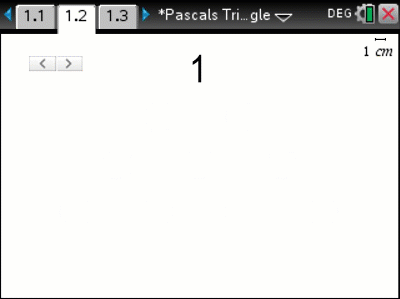•• ##### Device
•TI-Nspire™ CX CAS
• ##### Software

TI-Nspire™ CAS

4.5

# TAS: Pascals Triangle Hidden Gems

by Texas Instruments#### Objectives

By exploring Pascal’s triangle in greater depth, students are more likely to remember this amazing triangle. The numeric and algebraic applications will help improve students ‘by-hand’ algebraic skills beyond basic simplification.

#### Vocabulary

• Pascal
• Combinatorics
• Binomial Expansion
• Triangular Numbers
• Tetrahedral Numbers
• Fibonacci
• Euler
• Summation (Sigma Notation)
• Product (Pi Notation)

#### About the Lesson

Watch Activity Tutorial

Students make use of visual representations to help understand the basic construction of Pascal’s triangle and are then introduced to a plethora of other patterns within this amazing triangle. Students use an array of numeric and algebraic techniques to verify the patterns.